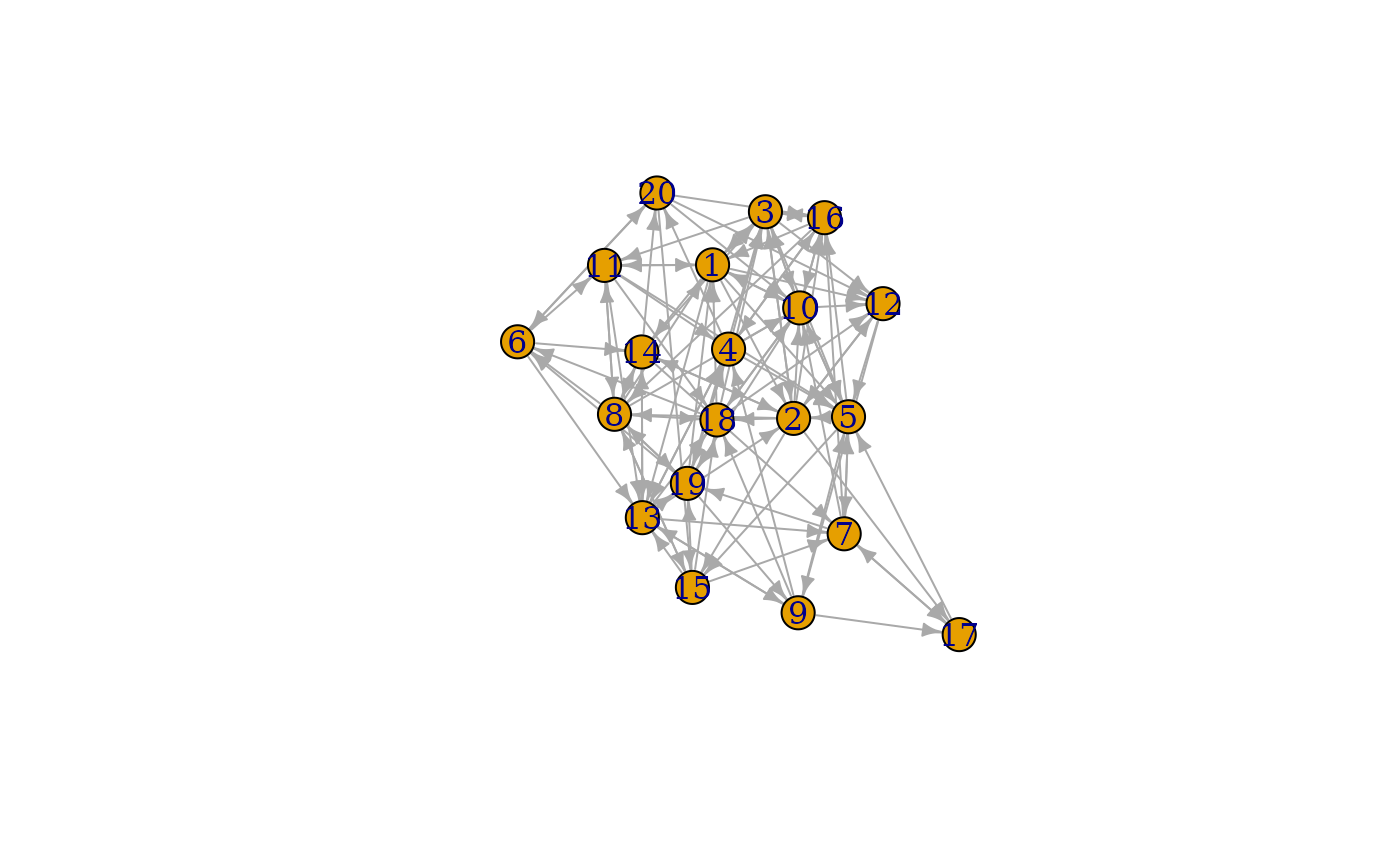This set of graph games creates graphs directly through sampling of different attributes, topologies, etc. The nature of their algorithm is described in detail at the linked igraph documentation.

play_degree(out_degree, in_degree = NULL, method = "simple")

play_dotprod(position, directed = TRUE)

play_fitness(m, out_fit, in_fit = NULL, loops = FALSE, multiple = FALSE)

play_fitness_power(
n,
m,
out_exp,
in_exp = -1,
loops = FALSE,
multiple = FALSE,
correct = TRUE
)

play_gnm(n, m, directed = TRUE, loops = FALSE)

play_gnp(n, p, directed = TRUE, loops = FALSE)

play_erdos_renyi(n, p, m, directed = TRUE, loops = FALSE)

## Arguments

out_degree, in_degree

The degrees of each node in the graph

method

The algorithm to use for the generation. Either 'simple', 'vl', or 'simple.no.multiple'

position

The latent position of each node by column.

directed

Should the resulting graph be directed

m

The number of edges in the graph

out_fit, in_fit

The fitness of each node

loops

Are loop edges allowed

multiple

Are multiple edges allowed

n

The number of nodes in the graph.

out_exp, in_exp

Power law exponent of degree distribution

correct

Use finite size correction

p

The probabilty of an edge occuring

The radius within which vertices are connected

torus

Should the vertices be distributed on a torus instead of a plane

## Value

A tbl_graph object

## Functions

• play_degree(): Create graphs based on the given node degrees. See igraph::sample_degseq()

• play_dotprod(): Create graphs with link probability given by the dot product of the latent position of termintating nodes. See igraph::sample_dot_product()

• play_fitness(): Create graphs where edge probabilities are proportional to terminal node fitness scores. See igraph::sample_fitness()

• play_fitness_power(): Create graphs with an expected power-law degree distribution. See igraph::sample_fitness_pl()

• play_gnm(): Create graphs with a fixed edge count. See igraph::sample_gnm()

• play_gnp(): Create graphs with a fixed edge probability. See igraph::sample_gnp()

• play_geometry(): Create graphs by positioning nodes on a plane or torus and connecting nearby ones. See igraph::sample_grg()

• play_erdos_renyi():Create graphs with a fixed edge probability or count. See igraph::sample_gnp() and igraph::sample_gnm()

Other graph games: component_games, evolution_games, type_games
plot(play_erdos_renyi(20, 0.3))
#> Warning: play_erdos_renyi() was deprecated in tidygraph 1.3.0.
#> ℹ Please use play_gnp() instead.